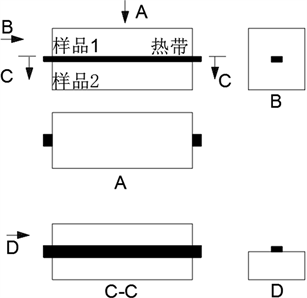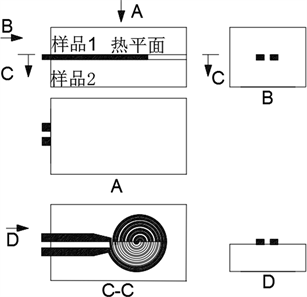# 瞬态法测量节能材料导热系数的研究Study on Measuring Thermal Conductivity of Energy-Saving Materials by Transient Method

• 全文下载: PDF(660KB)    PP.85-91   DOI: 10.12677/AEPE.2017.54014
• 下载量: 811  浏览量: 1,566   科研立项经费支持

The thermal conductivity is an important parameter that characterizes the low thermal conduc-tivity of energy-saving materials, and it is extremely important to measure it accurately. And the thermal conductivity with the energy-saving materials, composition, structure, phase and other changes, the measurement accuracy is the basis for the development of energy-saving materials. In this paper, the thermal conductivity of 12 kinds of energy-saving materials was measured and analyzed by means of transient tropical measurement device and transient plane heat source method. At the same time, the influence of each factor on the measurement results was analyzed. The method of transient measurement is used to verify the thermal conductivity of energy-saving materials.

1. 引言

2. 测量原理

2.1. 脉冲加热瞬态法

$\frac{\partial }{\partial x}\left(\lambda \frac{\partial T}{\partial x}\right)+\frac{\rho {I}^{2}}{{S}^{2}}-\frac{{\epsilon }_{h}\sigma P\left({T}^{4}-{T}_{0}^{4}\right)}{S}-\frac{\mu I}{S}\frac{\partial T}{\partial x}=\delta {c}_{p}\frac{\partial T}{\partial x}$ (1)

$\frac{\partial }{\partial x}\left(\lambda \frac{\partial T}{\partial x}\right)-\frac{{\epsilon }_{h}\sigma P\left({T}^{4}-{T}_{0}^{4}\right)}{S}=\delta {c}_{p}\frac{\partial T}{\partial x}$ (2)

$\lambda$ ——导热系数，W/(m∙K)；

$\rho$ ——电阻率，Ω∙m；

${\epsilon }_{h}$ ——全波长半球发射率；

$\mu$ ——托马逊因子；

$\delta$ ——密度，kg/m³；

${c}_{p}$ ——比热，J/(kg∙K)；

S——横截面积，m2

P——周长，m；

T——样品温度，K；

T0——环境温度，K。

2.2. 热线法

2.3. 探针法

2.4. 热带法

$R\left(t\right)=R\left[1+\alpha \Delta T\left(t\right)\right]$ (3)

$R\left(\tau \right)={R}_{0}\left[1+\left(\alpha {P}_{0}/\left(4{\text{π}}^{1/2}h\lambda \right)\right)f\left(\tau \right)\right]$ (4)

$f\left(\tau \right)=\tau \text{erf}\left(\frac{1}{\tau }\right)-\frac{{\tau }^{2}}{2\sqrt{\text{π}}\left[1-\mathrm{exp}\left(-{\tau }^{-2}\right)\right]}-\frac{1}{2\sqrt{\text{π}}}\left[Ei\left(-{\tau }^{-2}\right)\right]$ (5)

$\tau ={\left(kt/{d}^{2}\right)}^{1/2}$ (6)

R0——瞬时记录开始前探头电阻值，Ω；

Α——探头电阻温度系数，ppm/K；

ΔT(t)——探头温度随时间变化的函数，K；

P0——电源总输出功率，W；

λ ——样品导热系数，W/(m∙K)；

2h——热带长度，m；

f(τ)——假定平面热源厚度，m；

t——从瞬态开始记录的时间，s；

τ——无量纲参数

k——样品的热扩散系数，m2/s；

Ei——指数幂积分函数；

Erf——相对误差函数。

2.5. 热盘法

2.6. 瞬态平面热源法(TPS)

$R\left(t\right)=R\left[1+\alpha \Delta T\left(t\right)\right]$ (7)

$\Delta T\left(\tau \right)={P}_{0}{\left({\text{π}}^{3/2}\alpha \lambda \right)}^{-1}D\left(\tau \right)$ (8)

$D\left(\tau \right)={\left[m\left(m+1\right)\right]}^{2}{\int }_{0}^{\tau }{\sigma }^{-2}\left[{\sum }_{l=1}^{m}l{\sum }_{k=1}^{m}k\mathrm{exp}\left(\frac{-\left({l}^{2}+{k}^{2}\right)}{4{m}^{2}{\sigma }^{2}}\right){I}_{0}\left(\frac{lk}{2{m}^{2}{\sigma }^{2}}\right)\right]\text{d}\sigma$ (9)

$\tau ={\left(t/\theta \right)}^{1/2}={\left(t/\left({\alpha }^{2}/k\right)\right)}^{1/2}={\left(kt/{\alpha }^{2}\right)}^{1/2}$ (10)

R0——瞬时记录开始前探头电阻值，Ω；

Α——探头电阻温度系数，ppm/K；

△T(t)——探头温度随时间变化的函数，K；

P0——电源总输出功率，W；

λ——样品导热系数，W/(m∙K)；

α——传感器半径，m；

I0——瞬时起始电流值，A；

T——从瞬态开始记录的时间，s；

θ——特征时间，s；

τ——无量纲参数；

k——样品的热扩散系数，m2/s。

2.7. 双螺旋探针平面热源法(HotDisk)

HotDisk法，使用一种薄层圆盘形双螺旋结构探针同时作为平面热源和温度传感器，放置于表面光洁平整的相同被测样品中间，探头通电后温度上升，探头热阻系数随温度变化而变化，测量温升及响应时间，得到热导率。

2.8. 激光法

3. 测量装置与影响因素分析

3.1. 瞬态热带法测量装置

3.2. 瞬态平面热源法测量装置Figure 1. Measuring equipment of transient tropical methodFigure 2. Measuring equipment of transient plane heat source methodTable 1. Measurement data of thermal conductivity of energy-saving materials

3.3. 影响因素

4. 实验结果与讨论

5. 结论

  Cezairliyan, A. (1979) Advance in Measurements of the Thermos-Physical Properties by Dynamic Techniques. High Pressure, High Temperature, 11, 9-27.  Righini, F., et al. (2000) Pulse Calorimetry at High Temperatures. Ther-mochimica Acta, 347, 93-102.  Cezairliyan, A., et al. (1990) Issues and Future Directions in Subsecond Thermo-physics Research. International Journal of Thermophysics, 11, 819-833.  戴景民, 范毅, 褚载祥. 脉冲加热测量材料热物性技术的综述与分析[J]. 计量学报, 2001, 22(4): 249-253.  于帆, 张欣欣. 热带法测量材料导热系数的实验研究[J]. 计量学报, 2005, 26(1): 27-29.  Gustafsson, S.E. (1979) Transient Hot-Strip Method for Sim-ultaneously Measuring Thermal Conductivity and Thermal Diffusivity of Solids and Fluids. Journal of Applied Physi-ology, 12, 1411-1422.  Gustafsson, S.E. (1991) Transient Plane Source Techniques for Thermal Conductivity and Thermal Diffusivity Measurements of Solid Materials. The Review of Scientific Instruments, 62, 797-804.  Gustafsson, S.E., Karawacki, E. and Chohan, M.A. (1986) Thermal Transport Studies of Electrically Conducting Materials Using the Transient Hot-Strip Technique. Journal of Applied Physics, 19, 727-735.  孟飞燕, 张玫, 马亿珠, 等. 瞬态平面热源法测试材料导热系数的影响因素分析[J]. 河南科技, 2013(4): 59-60.  周孑民, 向锡炎, 陈晓玲, 张忠霞. 保温材料热物性测试的实验及数值研究[J]. 热科学与技术, 2007, 6(4): 368-372.  王强, 戴景民, 何小瓦. Hotdisk探头热容对热导率测量的影响[J]. 吉林大学学报(工学版), 2009, 39(1): 164-168.  周平. 保温板导热系数检测数据影响因素、偏差分析[J]. 建筑工程, 2013(10): 78.